IF YOU CAN SEE THIS go to /sfa/Nuprl/Shared/Xindentation_hack_doc.html

### Guarded Types: T(given P)

Sometimes when describing the intended types of arguments to functions, we want to hedge our typing with some further condition.

For example, the conjunction operator "A & B" of Nuprl is actually what is often called a "cand" or "conditional and", in which the second conjunct is required to be sensible only when the first conjunct is true. Consider the theorem:

Thm*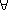k: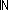. 0<k & (2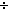k)<1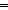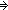2<k

Even if the expression "2k" is assigned no truth value for k = 0, it can occur in a context entailing k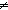0, such as in "0<k & (2k)<1" in this case.

The well-formedness of (conditional) "and" can be expressed thus:

Thm*A:Prop, B:Prop(given A). (A & B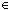Prop

Similarly, "AB" and even "B(given A)" itself are of the same kind.

Thm*A:Prop, B:Prop(given A). (ABProp

Thm*A:Prop, B:Type(given A). B(given AType

Thus, one can naturally express dependent propositions such as "k:. 0<k(2k)<1", where the truth of the antecedent would entail that the consequent makes sense.

More specifically, the membership of a type "T(given A)" has these properties: for any (closed) expression "e", if "A" is true then eT(given A) just when eT. If "A" is false then eT(given A) unconditionally.

There is a special connection between guarded types and Comprehension Subtypes:

Thm*P:(A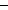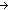Prop), B:(x:AType(given P(x))).
Thm* x:{u:AP(u) }B(x) =ext x:AB(x)(given P(x))